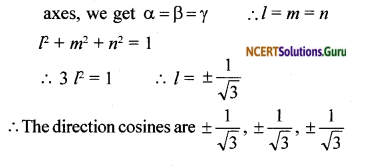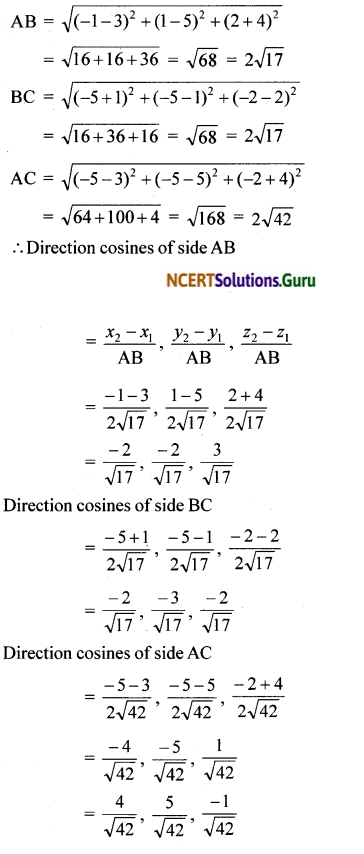# NCERT Solutions for Class 12 Maths Chapter 11 Three Dimensional Geometry Ex 11.1

These NCERT Solutions for Class 12 Maths Chapter 11 Three Dimensional Geometry Ex 11.1 Questions and Answers are prepared by our highly skilled subject experts.

## NCERT Solutions for Class 12 Maths Chapter 11 Three Dimensional Geometry Exercise 11.1Question 1.
If a line makes angles 90°, 135°, 45° with the x, y and z-axes respectively, find its direction cosines.
Solution:
Let the direction angles be α, ß, γ.
i.e., α = 90°, ß = 135° and γ = 45°
The direction cosines are cos α, cos ß, cos γ = cos 90°, cos 135°, cos45°
= 0, $$\frac{1}{\sqrt{2}}, \frac{1}{\sqrt{2}}$$

Question 2.
Find the direction cosines of a line which makes equal angles with the coordinate axes.
Solution:
Let the direction angles be α, ß, γ. Angles of the line. Since this makes equal angles with the axes, we getQuestion 3.
If a line has the direction ratios – 18, 12, – 4 then what are its direction cosines?
Solution:
The direction ratios are – 18, 12, – 4
$$\sqrt{(-18)^{2}+(12)^{2}+(-4)^{2}}=\sqrt{384}$$ = 22
The direction cosines are
$$\frac{-18}{22}, \frac{12}{22}, \frac{-4}{22}=\frac{-9}{11}, \frac{6}{11}, \frac{-2}{11}$$Question 4.
Show that the points (2, 3, 4) (- 1, – 2, 1), (5, 8, 7) are collinear.
Solution:
Let A (2, 3, 4), B (- 1, – 2, 1) and C (5, 8, 7) be the points.
Direction ratios of AB
= – 1 – 2, – 2 – 3, 1 – 4
= – 3, – 5, – 3
The direction ratios of BC
= 5 + 1, 8 + 2, 7 – 1 = 6, 10, 6
The direction ratios of AB and BC are proportional
∴ AB and BC are parallel.
Hence A, B, C are collinear.

Question 5.
Find the direction cosines of the sides of the triangle whose vertices are (3, 5, – 4), (- 1, 1, 2) and (- 5, – 5,- 2).
Solution:
Let A (3, 5, – 4) B (- 1, 1, 2) and C (- 5, – 5, – 2) are the vertices of ∆ABCerror: Content is protected !!